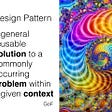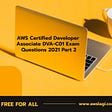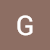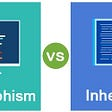# Complexity Classes & Its Types

• The problem is whether a given number is odd (or even).
• The problem is whether a given number is a prime number.
• The problem is whether a given number is in a specified finite or cofinite subset of natural numbers.
• The halting problem (whether a specified Turing machine halts or runs forever).
• The busy beaver problem (determining the length of the longest halting computation among Turing machines of a specified size).
• Rice’s theorem states that for all non-trivial properties of partial functions, it is undecidable whether a machine computes a partial function with that property.
• A finite set of tape symbols (input symbols and a blank symbol)
• A finite set of states
• A transition function

# P

• P is often taken to be the class of computational problems that are “efficiently solvable” or “tractable“.
• Problems that are solvable in theory, but cannot be solved in practice, are called intractable.
• There exist problems in P which are intractable in practical terms; for example, some require at least n1000000 operations.
• P is known to contain many natural problems, including the decision versions of linear programming, calculating the greatest common divisor, and finding a maximum matching.

# NP

• the Boolean satisfiability problem (SAT)
• the Hamiltonian path problem (a special case of TSP)
• the Vertex cover problem.
• Every decision problem that is solvable by a deterministic polynomial-time algorithm is also solvable by a polynomial-time non-deterministic algorithm.
• All problems in P can be solved with polynomial-time algorithms, whereas all problems in NP — P are intractable.
• It is not known whether P = NP. However, many problems are known in NP with the property that if they belong to P, then it can be proved that P = NP.
• If P ≠ NP, there are problems in NP that are neither in P nor in NP-Complete.
• The problem belongs to class P if it’s easy to find a solution for the problem. The problem belongs to NP, if it’s easy to check a solution that may have been very tedious to find.

# NP-Complete

• Approximation
• Probabilistic
• Special cases
• Heuristic
• Boolean satisfiability problem (SAT)
• N­puzzle
• Knapsack problem
• Hamiltonian cycle problem
• Traveling salesman problem
• Subgraph isomorphism problem
• Subset sum problem
• Clique problem
• Vertex cover problem
• Independent set problem
• Graph coloring problem
• Minesweeper
• Satisfiable: If the Boolean variables can be assigned values such that the formula turns out to be TRUE, then we say that the formula is satisfiable.
• Unsatisfiable: If it is not possible to assign such values, then we say that the formula is unsatisfiable.
• 2 edges for every clause i.e. ‘2m’ edges.
• 1 node for every Boolean variable involved in the Boolean formula.

# NP-Hard

1. If we can solve this problem in polynomial time, then we can solve all NP problems in polynomial time.
2. If you convert the issue into one form to another form within the polynomial time.

--

--

--

Love podcasts or audiobooks? Learn on the go with our new app.

## One Form Tow Model## How to deploy Strapi with Postgresql on Google App engine## Architecting for Reliability Part 2 — Resiliency and Availability Design Patterns for the Cloud## Refactoring## 3 Tips on How to Dockerize your Java8 Web Application## How to setup JDBC Kafka connect on local machine?## AWS Certified Developer Associate DVA-C01 Exam Questions 2021 Part 2## Comparison: Inheritance & Polymorphism## PunkScape Chapter II: From## CS373 Spring 2022: Ruchi Bhalani Week 8